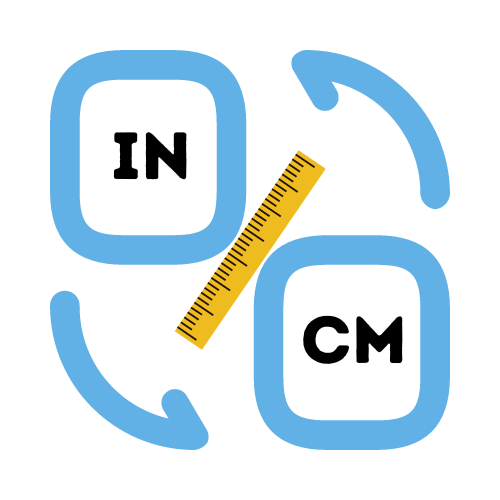# Convert CM to Inches

## Simple conversion of centimeters to inches.

• Created by Emily Rodriguez
• Reviewed by Ramesh Agarwal
Enter a number.

## How to convert centimeters to inches

Converting between centimeters and inches can be tricky when you're not used to it. There are lots of ways to convert both centimeters and inches, but some of them can be inaccurate. For example, sometimes it is better not to use a ruler when you're converting between units.

Ruler measurements are not always accurate, especially for smaller sizes, and this can lead to mistakes when you convert centimeters to inches. It is often easier and more accurate to use a calculator to convert between centimeters and inches.

It's important to understand the units and how they relate to each other. A centimeter has a length of 0.393701 inches. Knowing this, we can convert the size in both inches or centerimeters just by knowing the length in either cm or inches. A simple example of how you would convert 10cm to inches: 10 cm to inches can be calculated as 10 / 2.54 = 3.94 inches.

## What is the cm to inches formula?

Converting centimeters to inches can be a quick and easy process. Before you start, it is helpful to know the formula for converting between centimeters and inches.

If you know the value in inches, then you can use the following formula: inches / 2.54 = centimeters. If you know the length in centimeters and want to convert to inches, then you just need to take the number of centimeters and multiply it by 0.39.

You may use a calculator to do the above calculations once you know the cm to inches formula. However, if you don't have a calculator, you may use a pencil and paper.

As you can see, both centimeters and inches are used for measuring length in the metric and imperial systems. Both of these measurement systems are widely used across the world, but the two different systems can be confusing sometimes, especially when you're trying to convert from one to another.

## Benefits of using a conversion chart

Another way to do cm to inches conversion is to use a conversion chart. Conversion charts are very useful, and it means you don't need to do any calculations at all. Here's a handy conversion chart to help you convert cms to inches.

A conversion chart is great for conversions that are larger than a couple of centimeters. It is also good for conversions that involve fractions of centimeters. You can use a conversion chart to convert centimeters to inches if you need a quick conversion and don't have a calculator or unit converter available.

## Easy cm to inches conversion chart

Converting between the metric and imperial system can be tricky at times, especially when you don’t have a ruler to hand. That’s where our handy-dandy conversion chart comes into play. Let's break down some common measurements in centimeters, and the equivalent length in inches.

• 1 cm to inches = 0.39 inches
• 2 cm to inches = 0.79 inches
• 3 cm to inches = 1.18 inches
• 4 cm to inches = 1.57 inches
• 5 cm to inches = 1.97 inches
• 10 cm to inches = 3.94 inches
• 20 cm to inches = 7.87 inches
• 30 cm to inches = 11.81 inches
• 40 cm to inches = 15.75 inches
• 50 cm to inches = 19.69 inches

## How to use the cm to inches converter

Add the length you want to measure in centimeters and the cm to inch converter will give you the equivalent length in inches. This enables you to get quick and easy results without a calculator. You can also convert inches to centimeters by entering the value in inches in the same way.

If you want to convert cm to feet and inches, then you can use the cm to inches and feet calculator. These calculators will make it easy to do the length conversion for you, without needing to use a calculator or remember any difficult formulas.